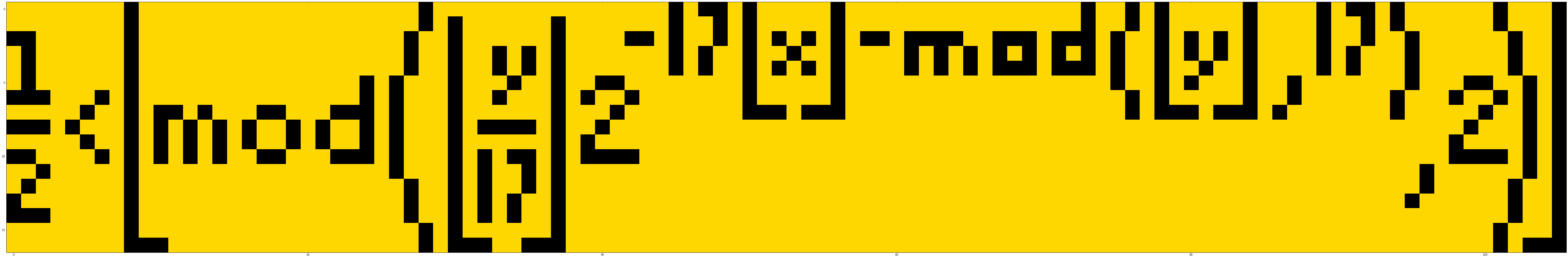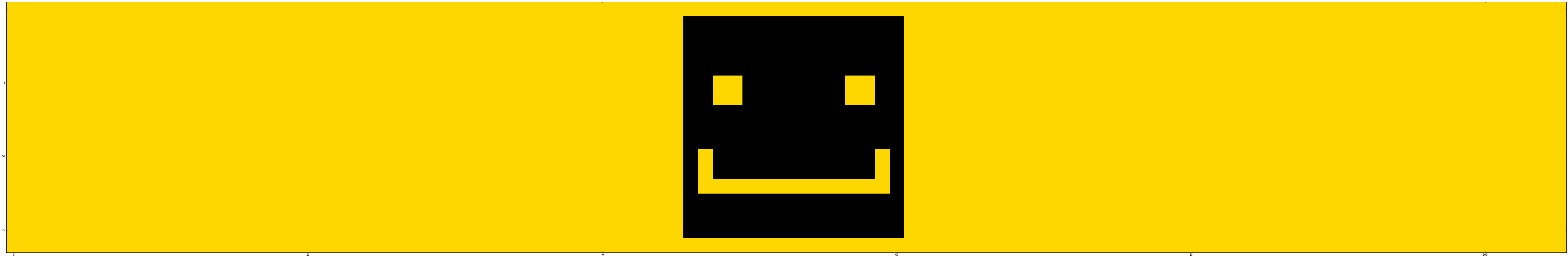# My résumé as a plot of a mathematical formula

All the pixel art you see in the jupyter notebook which serves as my résumé has been made by plotting Tupper's self-referential formula using matplotlib.

## Tupper's self-referential formula

If one plots the set of points (x, y) in 0 ≤ x < 106 and k ≤ y < k + 17 satisfying the inequality given below:

$$\displaystyle \frac12 < \left\lfloor \mathrm{mod} \left( \left\lfloor{\frac{y}{17}}\right\rfloor 2^{-17\lfloor x \rfloor - \mathrm{mod}(\lfloor y \rfloor, 17)}, 2 \right) \right\rfloor$$

where k is the following 543-digit integer:

In :
k = 960939379918958884971672962127852754715004339660129306651505519271702802395266424689642\
8421743507181212671537827706233559932372808741443078913259639413377234878577357498239266297\
1551717371699516523289053822161240323885586618401323558513604882869333790249145422928866708\
1096184496091705183454067827731551705405381627380967602565625016981482083418783163849115590\
2256100036523513703438744618483787372381982248498634650331594100549747005931383392264972494\
61751545728366702369745461014655997933798537483143786841806593422227898388722980000748404719


The resulting plot looks like this:

In :
%matplotlib inline
import matplotlib.pyplot as plt
import pandas as pd
import numpy as np
from numpy import vectorize
from matplotlib.colors import LinearSegmentedColormap

def plot(k):
@vectorize
def tupper(x,y):
return (y // 17 // 2 ** (17 * int(x) + int(y) % 17)) % 2 > 0.5
custom_cmap = LinearSegmentedColormap.from_list('taxi_driver', ['gold','black'])
X, Y = np.meshgrid(range(106), range(k, k + 17))
Z = np.fliplr(tupper(X, Y))
plt.figure(figsize = (106,17))
plt.imshow(Z, interpolation='nearest', cmap = custom_cmap)
plt.show()

plot(k)Look at the plot above and now have a look at the inequality again:

$$\displaystyle \frac12 < \left\lfloor \mathrm{mod} \left( \left\lfloor{\frac{y}{17}}\right\rfloor 2^{-17\lfloor x \rfloor - \mathrm{mod}(\lfloor y \rfloor, 17)}, 2 \right) \right\rfloor$$

We have visually reproduced the formula itself by plotting it. This is the beauty of Tupper's self-referential formula.

## Let's paint with numbers

Tupper's self-referential formula works by encoding 106 pixels wide by 17 pixels high bitmap in the constant k, and thus can be used to produce any 106 × 17 bitmap by finding the corresponding constant k. The algorithm for finding the constant k for a given 106 × 17 bitmap is:

Treat the bitmap as a 106 × 17 grid and place a 1 in the squares you want to be black and a 0 in the squares you want to be white, rotating the grid and reading the digits off left to right, working down the grid, will give you a 1802-digit binary number. Convert that number into base-10, then multiply it by 17.

I first draw my pixel art in a 106 × 17 canvas in Hexels 2 (my tool of choice for pixel art), then I export it as csv. The format of csv is:

(column), (row), (R), (G), (B), (A)

I read the corresponding csv, convert it into a numpy array and apply the algorithm mentioned above to find the constant k:

In :
matrix = np.zeros(shape = (17,106))
binary_string = ""

for _index, row in df[4:].iterrows():
point = list(map(lambda x: x.strip(), row.split(',')))
if((point, point, point, point) != ("0", "0", "0", "0")):
matrix[int(point)][int(point)] = 1

matrix = np.flipud(matrix).transpose()
for x in np.nditer(matrix, order = 'C'):
binary_string = binary_string + str(int(x))
k = int(binary_string, 2) * 17
print(k)

95500182201516581983920832671714565770426455565039891778737160107385569229269004793065306017186042923264556239058871166553957021501919629853468637788806704019867929944870261186178167391177949556483433038129626397447270622316936946925721496748773641203162582493261712599174437744370033500589089755659572871168

In :
plot(k)